Next: 2-var LP's with many Up: Graphical solution of 2-var Previous: Representing the Objective Function

#### Graphical solution of the prototype example: a 2-var LP with a unique optimal solution

The `` sliding motion'' described above suggests a way for identifying the optimal values for, let's say, a max LP problem. The underlying idea is to keep ``sliding'' the isoprofit line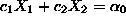in the direction of increasing's, until we cross the boundary of the LP feasible region. The implementation of this idea on the prototype LP of Equation 5 is depicted in Figure 3.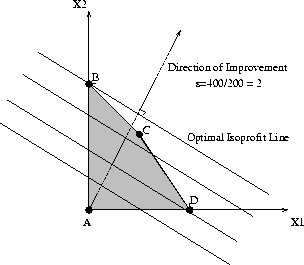Figure 3: Graphical solution of the prototype example LP

From this figure, it follows that the optimal daily production levels for the protoype LP are given by the coordinates of the point corresponding to the intersection of line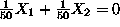with the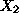-axis, i.e.,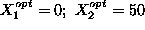. The maximal daily profit is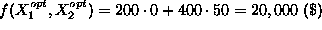. Notice that the optimal point is one of the ``corner'' points of the feasible region depicted in Figure 3. Can you argue that for the geometry of the feasible region for 2-var LP's described above, if there is a bounded optimal solution, then there will be one which corresponds to one of the corner points? (This argument is developed for the broader context of n-var LP's in the next section.)

UAL Data
Fri Jun 20 15:03:05 CDT 1997